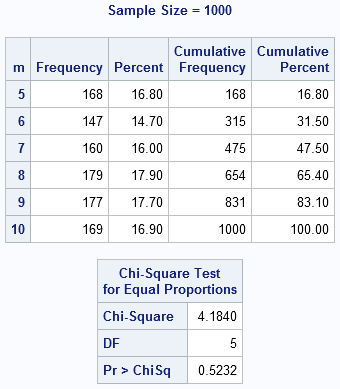# How to generate random numbers in SAS

August 24, 2011
By

 This post was kindly contributed by The DO Loop - go there to comment and to read the full post.

In SAS, you can generate a set of random numbers that are uniformly distributed by using the RAND function in the DATA step or by using the RANDGEN subroutine in SAS/IML software. (These same functions also generate samples from other common distributions such as binomial and normal.)
The syntax is simple. The following DATA step creates a data set that contains 10 random uniform numbers in the range [0,1]:

``` data A; call streaminit(123); /* set random number seed */ do i = 1 to 10; u = rand("Uniform"); /* u ~ U[0,1] */ output; end; run; ```

The syntax for the SAS/IML program is similar, except that you can avoid the loop (vectorize) by allocating a vector and then filling all elements by using a single call to RANDGEN:

``` proc iml; call ranseed(123); /* set random number seed */ u = j(10,1); /* allocate */ call randgen(u, "Uniform"); /* u ~ U[0,1] */ ```

### Random uniform on the interval [a,b]

If you want generate random numbers on the interval [a,b], you have to scale and translate the values that are produced by RAND and RANDGEN. The width of the interval [a,b] is b-a, so the following statements produce random values in the interval [a,b]:

``` a = -1; b = 1; /* example values */ x = a + (b-a)*u; ```

The same expression is valid in the DATA step and the SAS/IML language.

### Random integers

You can use the FLOOR or CEIL functions to transform (continuous) random values into (discrete) random integers. In statistical programming, it is common to generate random integers in the range 1 to Max for some value of Max, because you can use those values as observation numbers (indices) to sample from data. The following statements generate random integers in the range 1 to 10:

``` Max = 10; k = ceil( Max*u ); /* uniform integer in 1..Max */ ```

If you want random integers between 0 and Max or between Min and Max, the FLOOR function is more convenient:

``` Min = 5; n = floor( (1+Max)*u ); /* uniform integer in 0..Max */ m = min + floor( (1+Max-Min)*u ); /* uniform integer in Min..Max */ ```

Again, the same expressions are valid in the DATA step and the SAS/IML language.

### Putting it all together

The following DATA step demonstrates all the ideas in this blog post and generates 1,000 random uniform values with various properties:

``` %let NObs = 1000; data Unif(keep=u x k n m); call streaminit(123); a = -1; b = 1; Min = 5; Max = 10; do i = 1 to &NObs; u = rand("Uniform"); /* U[0,1] */ x = a + (b-a)*u; /* U[a,b] */ k = ceil( Max*u ); /* uniform integer in 1..Max */ n = floor( (1+Max)*u ); /* uniform integer in 0..Max */ m = min + floor((1+Max-Min)*u); /* uniform integer in Min..Max */ output; end; run; ```

You can use the UNIVARIATE and FREQ procedures to see how closely the statistics of the sample match the characteristics of the populations. The PROC UNIVARIATE output is not shown, but the histograms show that the sample data for the u and x variables are, indeed, uniformly distributed on [0,1] and [-1,1], respectively. The PROC FREQ output shows that the k, n, and m variables contain integers that are uniformly distributed within their respective ranges. Only the output for the m variable is shown.

``` proc univariate data=Unif; var u x; histogram u/ endpoints=0 to 1 by 0.05; histogram x/ endpoints=-1 to 1 by 0.1; run;   proc freq data=Unif; tables k n m / chisq; run; ```This post was kindly contributed by The DO Loop - go there to comment and to read the full post.

Tags: , , ,

## Welcome!

SAS-X.com offers news and tutorials about the various SAS® software packages, contributed by bloggers. You are welcome to subscribe to e-mail updates, or add your SAS-blog to the site.Dear readers, proc-x is looking for sponsors who would be willing to support the site in exchange for banner ads in the right sidebar of the site. If you are interested, please e-mail me at: tal.galili@gmail.com